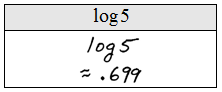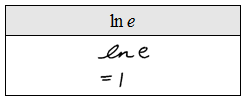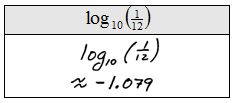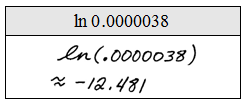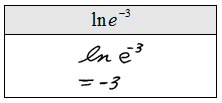## Algebra

Showing posts with label rules. Show all posts
Showing posts with label rules. Show all posts

### Logarithmic Functions

In the last section we made note of the fact that exponential functions pass the horizontal line test and thus have an inverse.

YouTube Playlist: Logarithms and their Graphs

Using the steps for finding the inverse we obtain:At this point there is no way to solve for y. Therefore we make the following definition:Here are some examples of logarithmic facts and their equivalent in exponential form.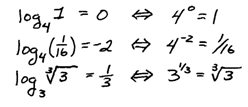Use this definition to rewrite the following in logarithmic form.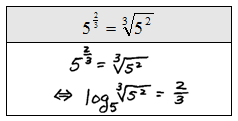Use the proper terminology when reading logarithms,reads “log base 5 of 125 is 3.”  If given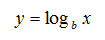then x is called the argument of the logarithm. Also, notice that y is an exponent so the logarithm is actually an exponent, this will be important later.

Evaluate without using a calculator.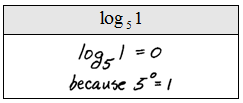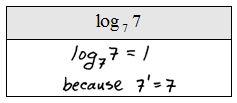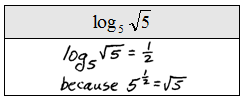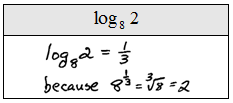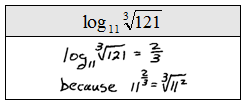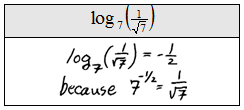Instructional Video: Introduction to Logarithms

The base b can be any real number greater than zero but not including one - 10 and the natural base e are used often.A logarithm without a base is interpreted as the common logarithm.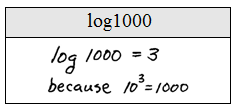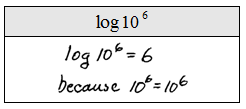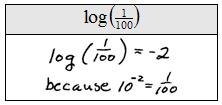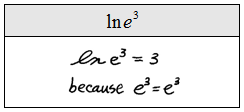Often the logarithms do not work out so nicely and we will need to use a calculator to evaluate them.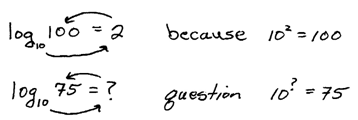Here are the steps for using a TI-30x calculator.  Other scientific calculators are similar.So if we round off the nearest thousandth we haveEvaluate using a calculator rounding off to the nearest thousandth.